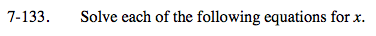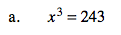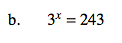Home > A2C > Chapter 7 > Lesson 7.2.3 > Problem7-133

7-133.
1. Solve each of the following equations for x. Homework Help ✎

1. x3 = 243

2. 3x = 243Use roots or fractional exponents.Use logarithms or find a common base.